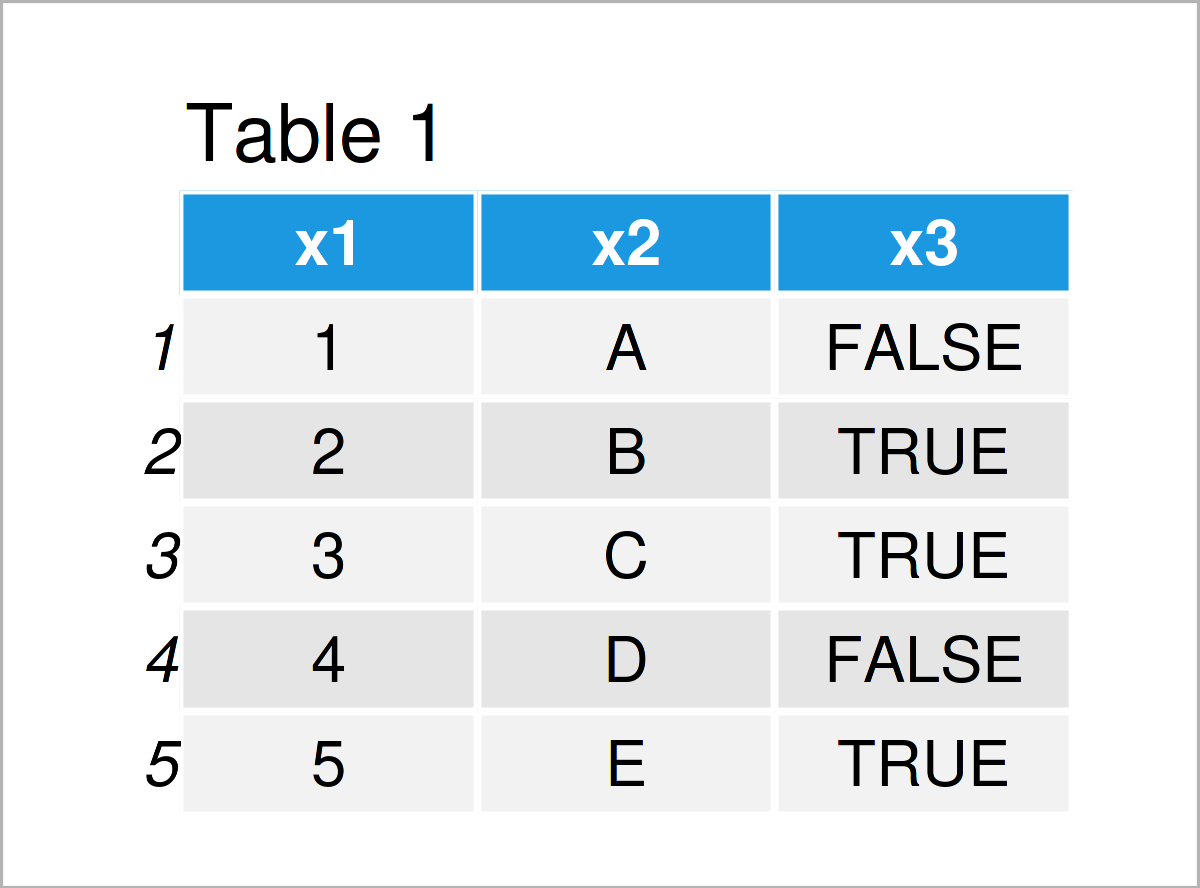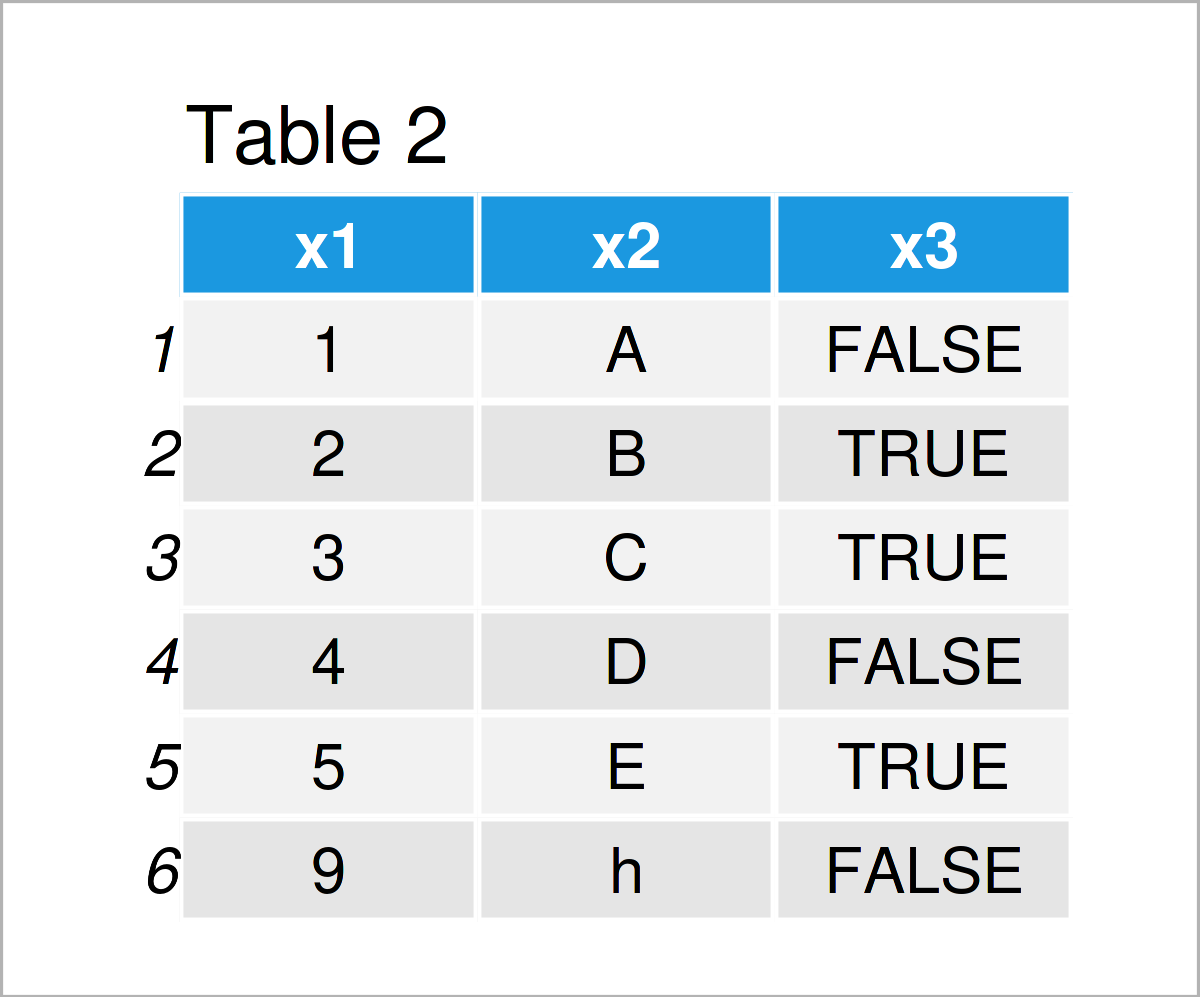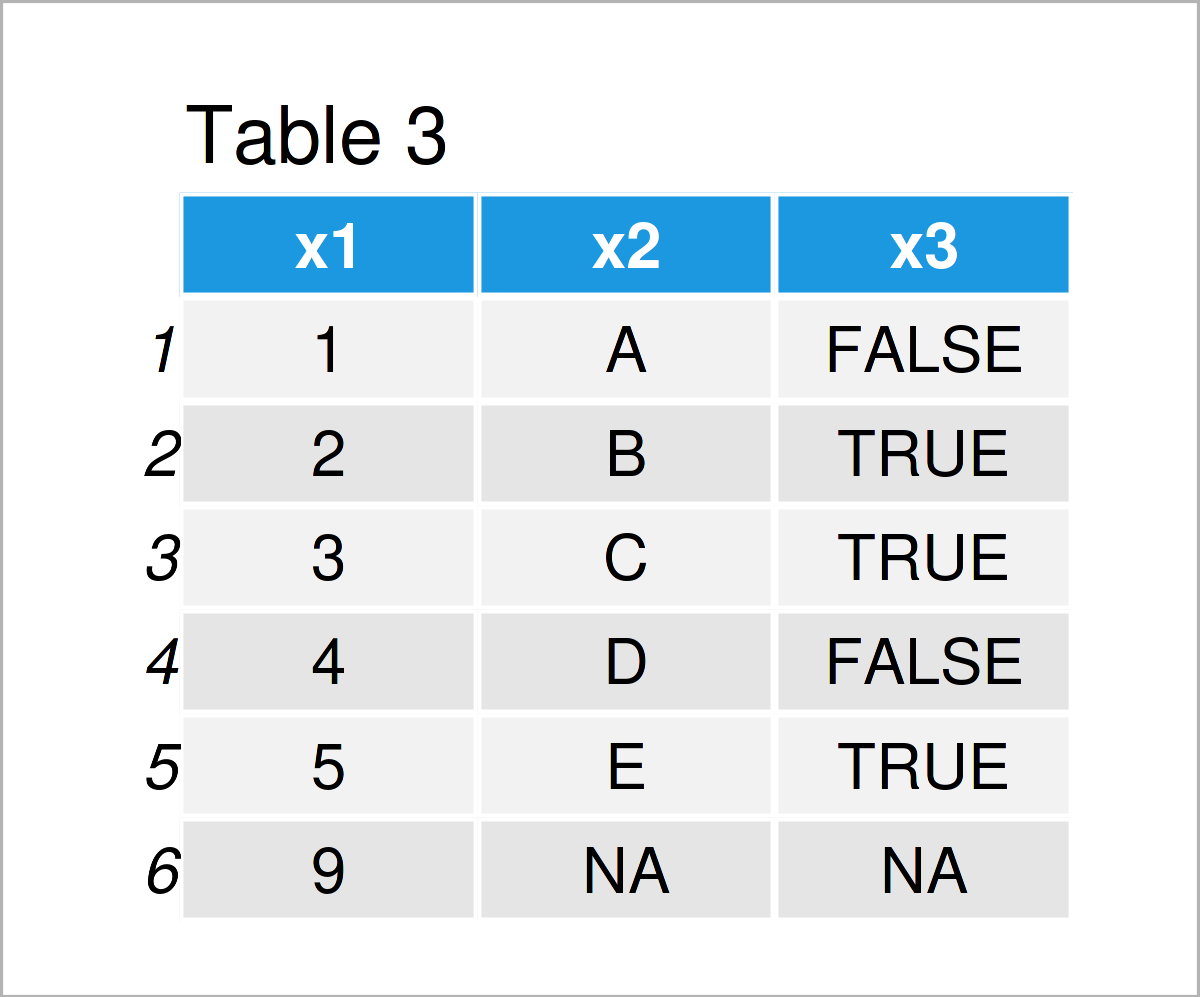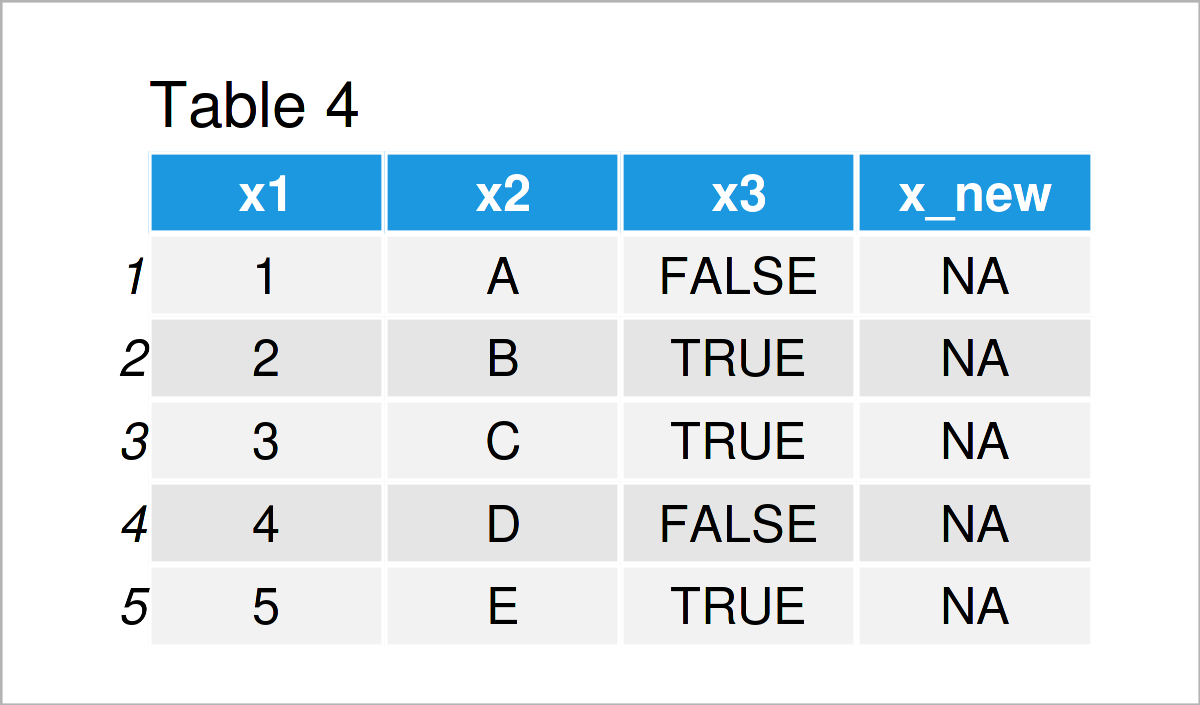# Add Row & Column to data.table in R (4 Examples)

This article explains how to combine a data.table with an additional row or column in the R programming language.

Let’s start right away:

## Example Data & Add-On Packages

We first have to install and load the data.table package:

```install.packages("data.table")                                   # Install data.table package

We use the following data as a basis for this R tutorial:

```dt_orig <- data.table("x1" = 1:5,
"x2" = LETTERS[1:5],
"x3" = c(FALSE, TRUE, TRUE, FALSE, TRUE))  # Create data.table
dt_orig```Table 1 shows the structure of our example data.table: It is constituted of five observations and three columns with integer, string, and logical input.

## Example 1: Add a Row

Example 1 shows how to merge a data.table with an additional row. For that, we first replicate dt_orig, called dt_add_row. The new row which we want to add to the data can be defined as an additional data.table object, called new_row. It corresponds to a data.table with only one row. The column types and names of new_row fit to those of dt_add_row. With command rbindlist from the data.table package, we can append dt_add_row and new_row row-wise.

```dt_add_row <- data.table::copy(dt_orig)                          # Replicate dt_orig
new_row    <- data.table("x1" = 9, "x2" = "h", x3 = FALSE)Object dt_add_row, shown in Table 2, shows the original data.table with the added row number 6.

## Example 2: Add a Row With Partially Missing Values

Now we add another row to a data.table, where we only have information on some, but not all columns. To put it differently, we want to append a new row where some information is missing. As with the example before, the new row is created as a data.table object, called new_row2. We can again use function rbindlist to combine the original data and new_row2 row-wise. Only now, we use the additional function argument “fill = TRUE”. With this argument, we say that all missing column information is filled by NAs.

```dt_add_row2 <- data.table::copy(dt_orig)                         # Replicate dt_orig
new_row2    <- data.table("x1" = 9)
fill = TRUE)                            # Missing columns are filled with NAThe result is shown in Table 3. The additional row number 6 only contains information on x1. There is no information on variables x2 and x3. The values for x2 and x3 are therefore filled by NAs when merging the row to dt_add_row2.

## Example 3: Add a Column

In this example, we add a new column to a data.table. In a data.table, we index rows and columns by square brackets, like data.table[ indexed rows, indexed columns ]. When we want to add a new column, we can simply use the following lines of code.

```dt_add_col <- data.table::copy(dt_orig)                          # Replicate dt_origIn Table 4 it is shown that have joined a new column x_new with the data.table using the previous R syntax. We simply assign NA to the values of the new column x_new.

## Example 4: Add New Column With String Object as Column Name

This example demonstrates how to merge a data.table with a new column, where the name of the column is stored as a string in an additional object. We have the object new_column_name which contains the name of the new column. To create a new column with that name, the R code shown below can be used.

```dt_add_col2     <- data.table::copy(dt_orig)                     # Replicate dt_orig
new_column_name <- "x_new"In Table 6 you can see that we have created a new column called x_new. We had to use round brackets to indicate that we are interested in the string stored in object new_column_name to name the new column.

## Video & Further Resources

Do you want to know more about the combining a data.table with an additional row or column? Then I recommend watching the following video on my YouTube channel. I illustrate the content of this tutorial in the video.

Furthermore, you may want to have a look at the related tutorials on my website. I have published several articles that are related to the combining of a data.table with an additional row or column already:

Summary: In this article, you have learned how to combine a data.table with an additional row or column in the R programming language. Kindly let me know in the comments, if you have further questions.

This page was created in collaboration with Anna-Lena Wölwer. Have a look at Anna-Lena’s author page to get more information about her academic background and the other articles she has written for Statistics Globe.

Subscribe to the Statistics Globe Newsletter